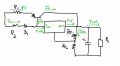# Voltage Regulator problem

#### PsySc0rpi0n

Joined Mar 4, 2014
1,706
Hello

I have this circuit to find the maximum output current that the circuit can "deliver" to the load!The info we have is:
R1 = 0.2Ω
R2 = 1Ω
R_F1 = 500Ω
R_F2 = 250Ω
C1 = 1μF

NPN
Ic max = 10 A
hFE = 50
|Vbe| = 0.7 V

7809
Iq = 5 mA
Io (short-circuit) = 1 A
Vdrop out min = 2 V

The first question was to find the output voltage for the circuit, knowing that Vin is an adequate DC value with 1 V of ripple!
I found this value to be ~14.75V

But now I can't find a way of evaluating the max output current that is the 2nd question!
I wrote an equation for the loop that includes the diode, R1, R2 and Veb as:

Ie*R1 + Veb -Vd1 - I1*R2 = 0

Veb and Vd1 cancels out, so:

Ic*R1*(hFE + 1) / hFE = I1*R2
I1 =(R1/R2)*Ic*((hFE + 1) / hFE)

Then I wrote:

Io' = Iin + Iq
Io' = (Ic/hFE + I1) + Iq
replace I1 by the above result, in terms of Ic
Io' = (Ic/hFE + (R1/R2)*Ic*((hFE + 1) / hFE)) + Iq

and:

Iout = Ic + Io' - I_RF1
Iout = Ic * (1/hFE + (hFE + 1) / hFE) * (R1/R2) + 1) - I_RF1 + Iq

but I end up with an expression with 2 unknowns, and I'm not getting how am I going to find Iout!

#### dannyf

Joined Sep 13, 2015
2,197
The 7809 will develop 9v on Rf1 and the output voltage would be (1 + Rf2 / Rf1) * (9v/Rf1).

You can answer the current question hopefully.

#### crutschow

Joined Mar 14, 2008
32,848
First, you have the output voltage wrong.
The output of the 7809 is 9V, giving a current through Rf1 of 9V / 500 = 18ma.
This current gives a voltage across Rf1 of 18ma * 250 = 4.5V.
The output voltage is then 9V + 4.5V = 13.5V.

Then calculate the current by assuming the transistor base current is zero. That will simplify the equations and should allow a single-equation solution, giving only a small error (for typical values of Beta).
After you find that value, you can go back a tweak it for the actual base current, if you need to.

#### MrAl

Joined Jun 17, 2014
10,587
Hi,

If there was no base current, there would be a balance when the voltages across both R1 and R2 were equal. From there you can get the currents, then later think about what the base current does.
The solution point for the current through R2 is the current limit of the IC, and with the base current included it will be less and you should be able to figure that out.

#### Jony130

Joined Feb 17, 2009
5,457
Notice that you know the 7806 short circuit current Io (short-circuit) = 1 A. So the voltage across R2 is1A*1Ω = 1V and this also means that the emitter current is equal to Ie ≈ 1V/0.2Ω ≈ 5A, So the load current is Iload ≈ 1A + 5A ≈ 6A
Or notice that I1 + Ib1 = 1.005A and additional one Ib1 = Ie/(β + 1) and now you can do your exact calculations.

Last edited:

#### atferrari

Joined Jan 6, 2004
4,699

#### Bordodynov

Joined May 20, 2015
3,084
Vout=9V + 4.5V+5mA*0.25kOhm=9V+4.5V+1.25V=14.75V !
5mA is curren from pin COM !
LM317 ==> curren from pin adj =50uA.

#### PsySc0rpi0n

Joined Mar 4, 2014
1,706
Hi guys... Thanks for all the replies!!!

Well, in my teacher's point of view, things are quite different!

@crutschow the voltage across RF1 is 9 V, making the current through it of 18 mA. But then the current flowing through RF2 is I_RF1 + IQ. So the voltage drop across RF2 = (18 mA + 5 mA)*250Ω = 5.75 V. So the Vout = 9 V + 5.75 V = 14.75 V.

But in my teacher's point of view is that ignoring Iq, Iin = Io', so Io' = I1 + Ib = 1 A. Also the net closed loop that includes R1, R2, D1 and Veb says that I1 = Ie * (R2/R1).

Io' = I1 + Ib

Replacing Ib by Ie/(hFE + 1), we get:

Ie = Io' / (R1 / R2 + 1/(hFE + 1))
Ie = 1 / (0.2/1 + 1/(50+1) )
Ie = 4.554 A

Ic = 4.554 / 50 = 4.464 A

Ignoring I_RF1, we get

Iout = Io' + Ic = 1 A + 4.464 A = 5.464 A

I think this was the correct result. If we included IQ and I_RF1 the result would be similar!